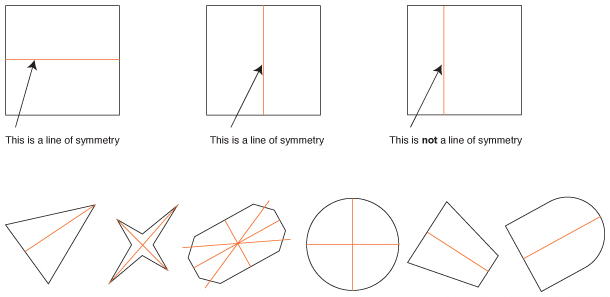# Stage 2 - space and geometry

## Strategy

Students can:

• identify various 2D shapes
• mark lines of symmetry in a variety of 2D shapes
• classify angles

## Activities to support the strategy

### Teaching strategy – examples and non-examples

Identification of shapes requires conceptual understanding. This is best taught by providing a definition of the concept along with examples and non-examples of the concept. Likewise conceptual understanding of symmetry is best taught by providing a definition followed by examples and non-examples of the concept.

When using the principles of ‘examples and non-examples’ to teach conceptual understanding start with non-examples that are very different from the target shape or concept then gradually include non-examples that differ only slightly from the target shape.

These questions require conceptual understanding that could be taught using examples and non-examples.

### Examples of teaching concepts by example and non-example

#### Concept of a circle

• Definition – a circle is a flat round shape with every point on its edge being the same distance from the middle.Variation: use the same approach to teach the concept of a cylinder and prism by providing a definition and then showing examples and non-examples of the concept.

#### Concept of a right angle

• Definition – a right angle is an internal angle which is equal to 90 degrees.Note: The special symbol like a box in the angle. If you see this, it is a right angle. The 90° is rarely written in. If you see the box in the corner, you are being told it is a right angle. This box does not always appear in a right angle.

All the angles below are right angles:A right angle can be in any orientation or rotation as long as the internal angle is 90°.

Make up a range of cards showing examples and non-examples of a right angle.

Have students respond to each card by saying “right angle” or “not a right angle”.

• Remember to start with non-examples that are quite different from a right angle and move to non-examples that are close to a right angle.
• Use the same strategy to teach concepts of acute angle and obtuse angle

#### Concept of a line of symmetryView/print examples of symmetry (PDF 183.53KB)

• Definition – a line of symmetry divides a shape so that each side of the line is exactly the same.
• Have students determine the lines of symmetry for a range of shapes.
• Have students fold shapes along a line that is NOT a line of symmetry.

## References

#### Australian curriculum reference

ACMMG088: Compare and describe two dimensional shapes that result from combining and splitting common shapes, with and without the use of digital technologies ACMMG066: Identify symmetry in the environment

#### NSW syllabus reference

MA2-15MG: Manipulates, identifies and sketches two-dimensional shapes, including special quadrilaterals, and describes their features.

MA2-16MG: Identifies, describes, compares and classifies angles